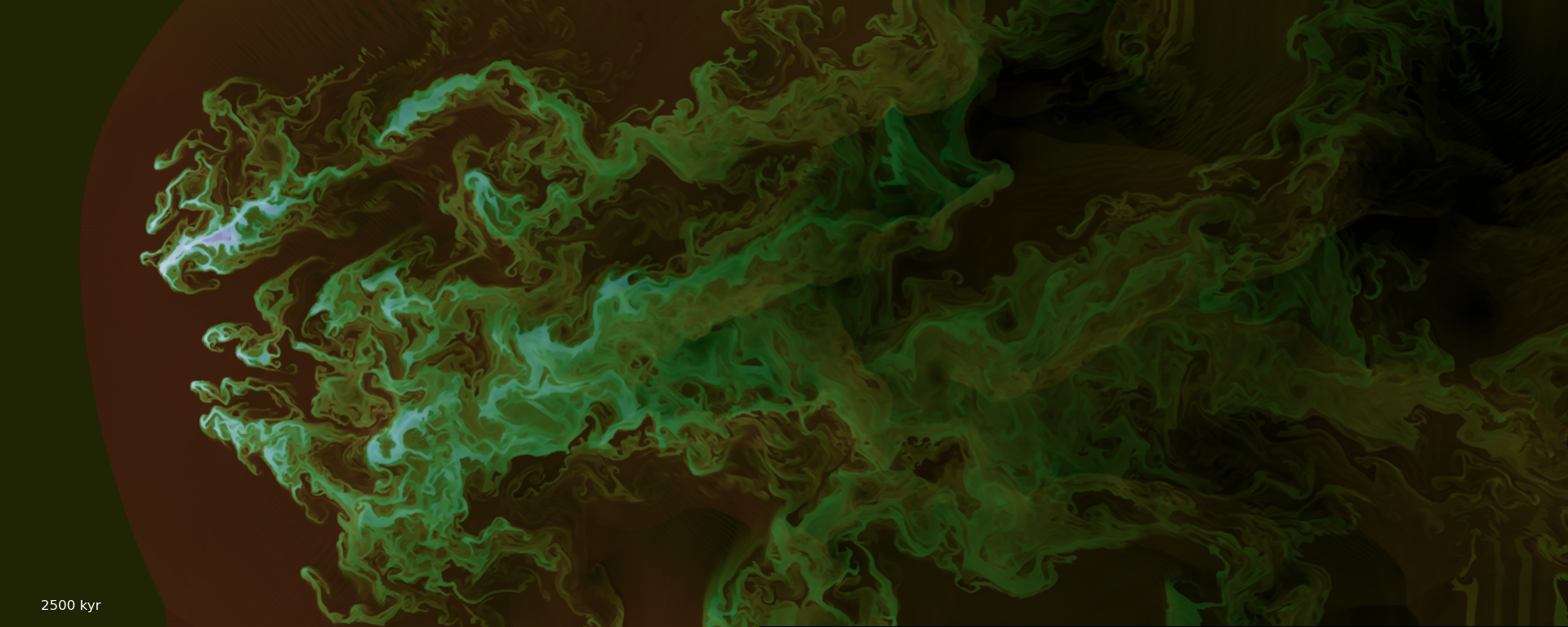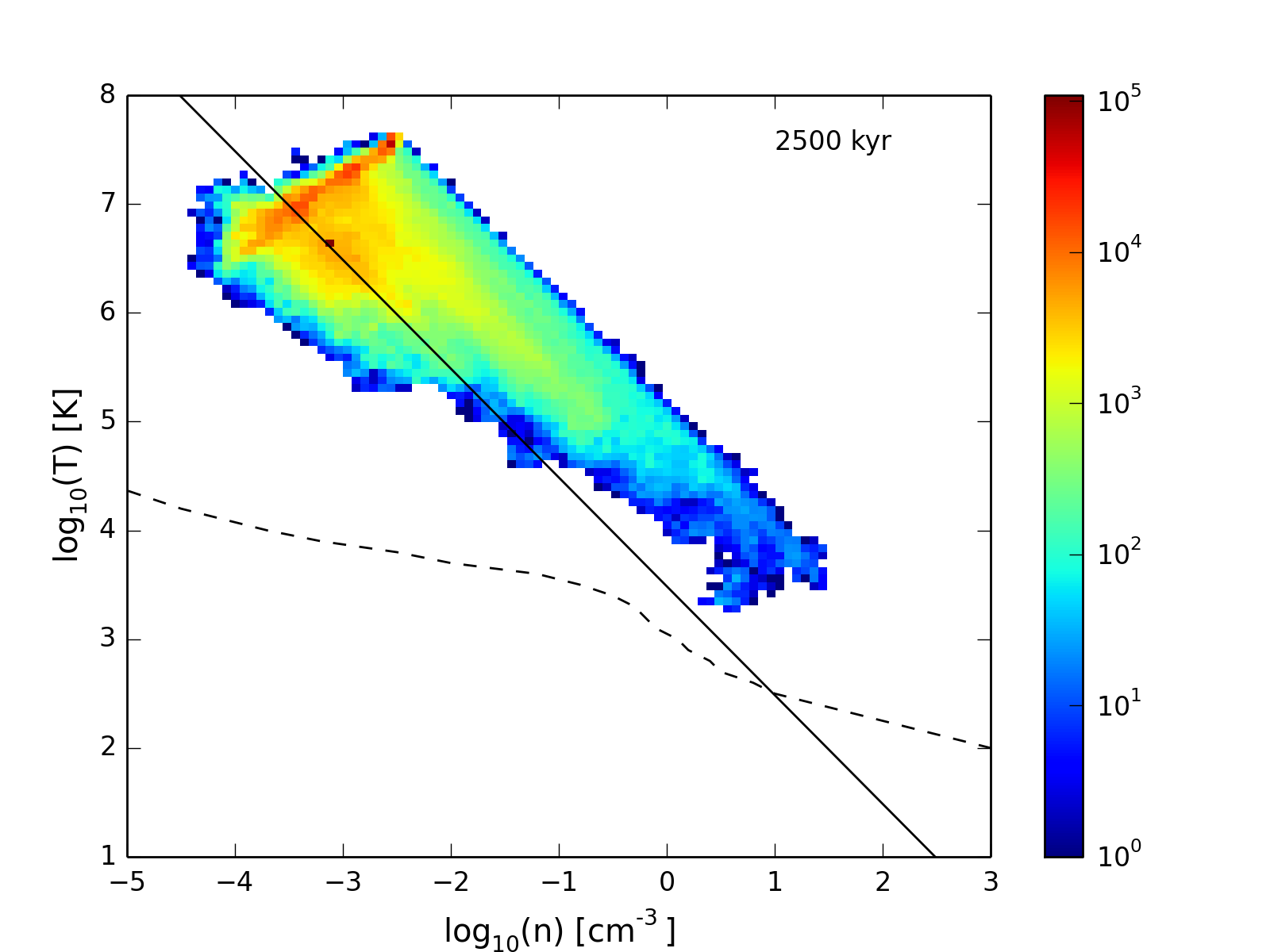Boundary Conditions

February 22, 2016

When high density shocked material gets too close to the simulation boundary, unphysical pressure gradients develop. The snapshot below shows an example of such behavior in a 3D simulation with $n_\mathrm{cloud} = 10$.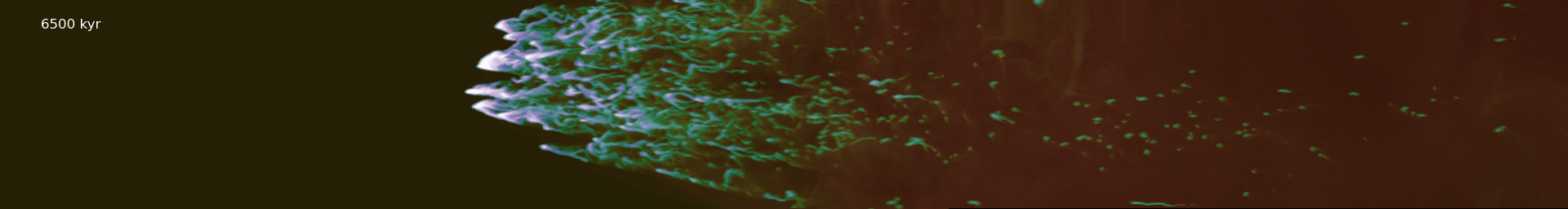This behavior manifests in the density-temperature phase diagram as a signifant fraction of gas that is not in pressure equilibrium with the hot wind: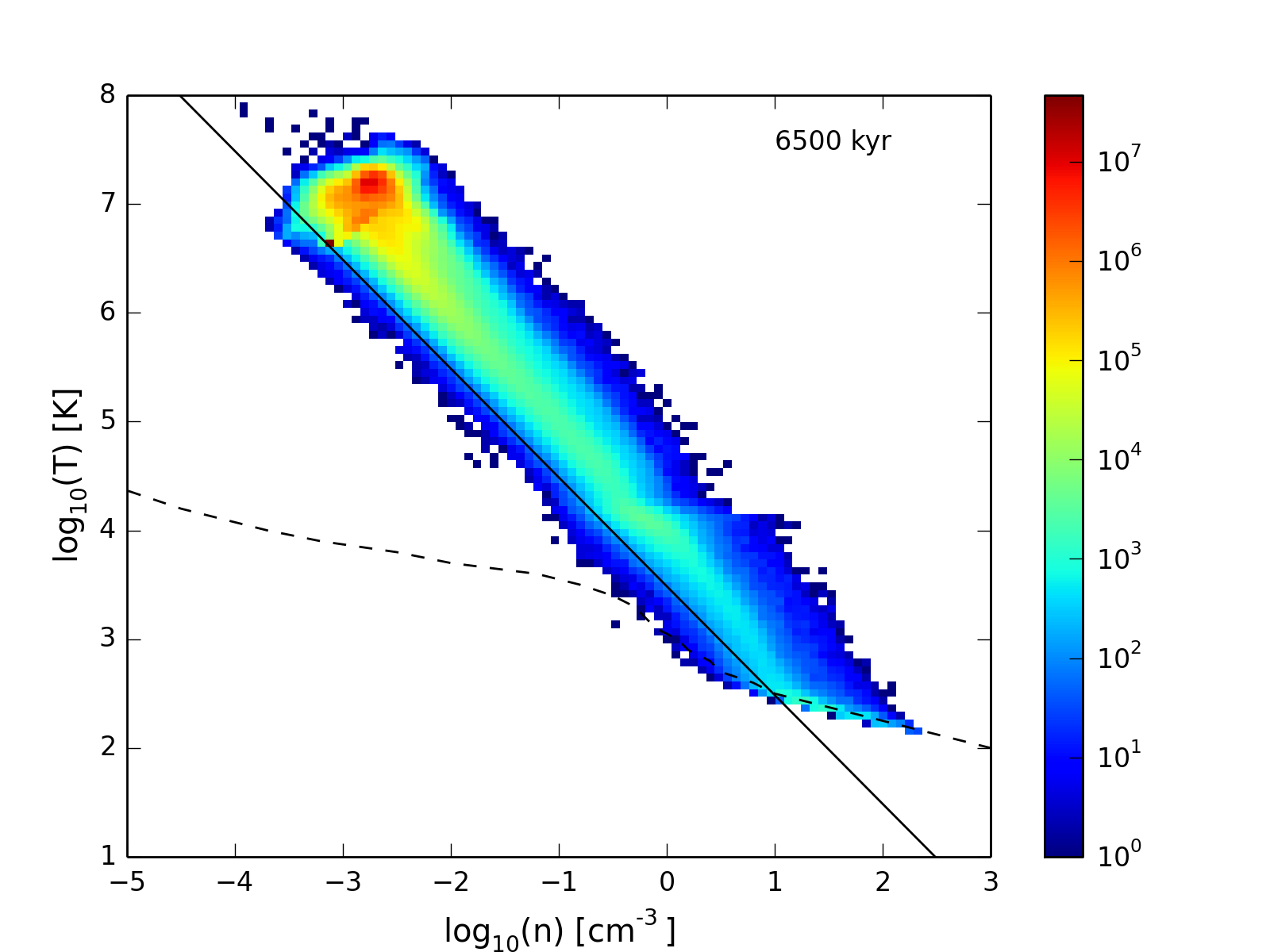To test what size simulations are needed to overcome the problem, we can run very large 2D simulations. First we check that the behavior shows up in 2D as well. The following simulation uses a 2D cloud with $n_\mathrm{cloud} = 1$.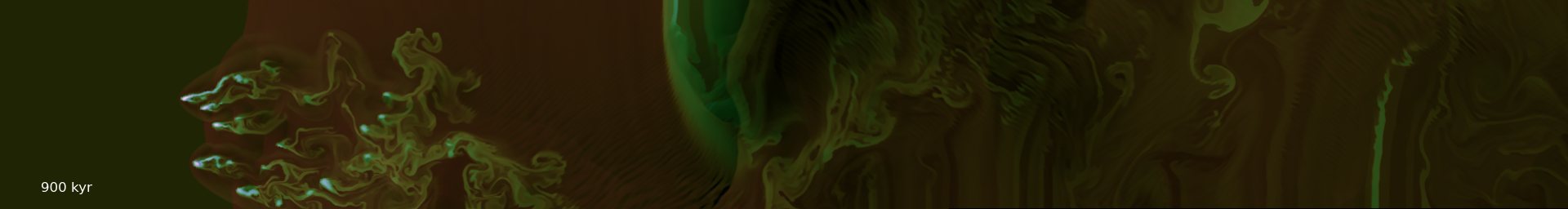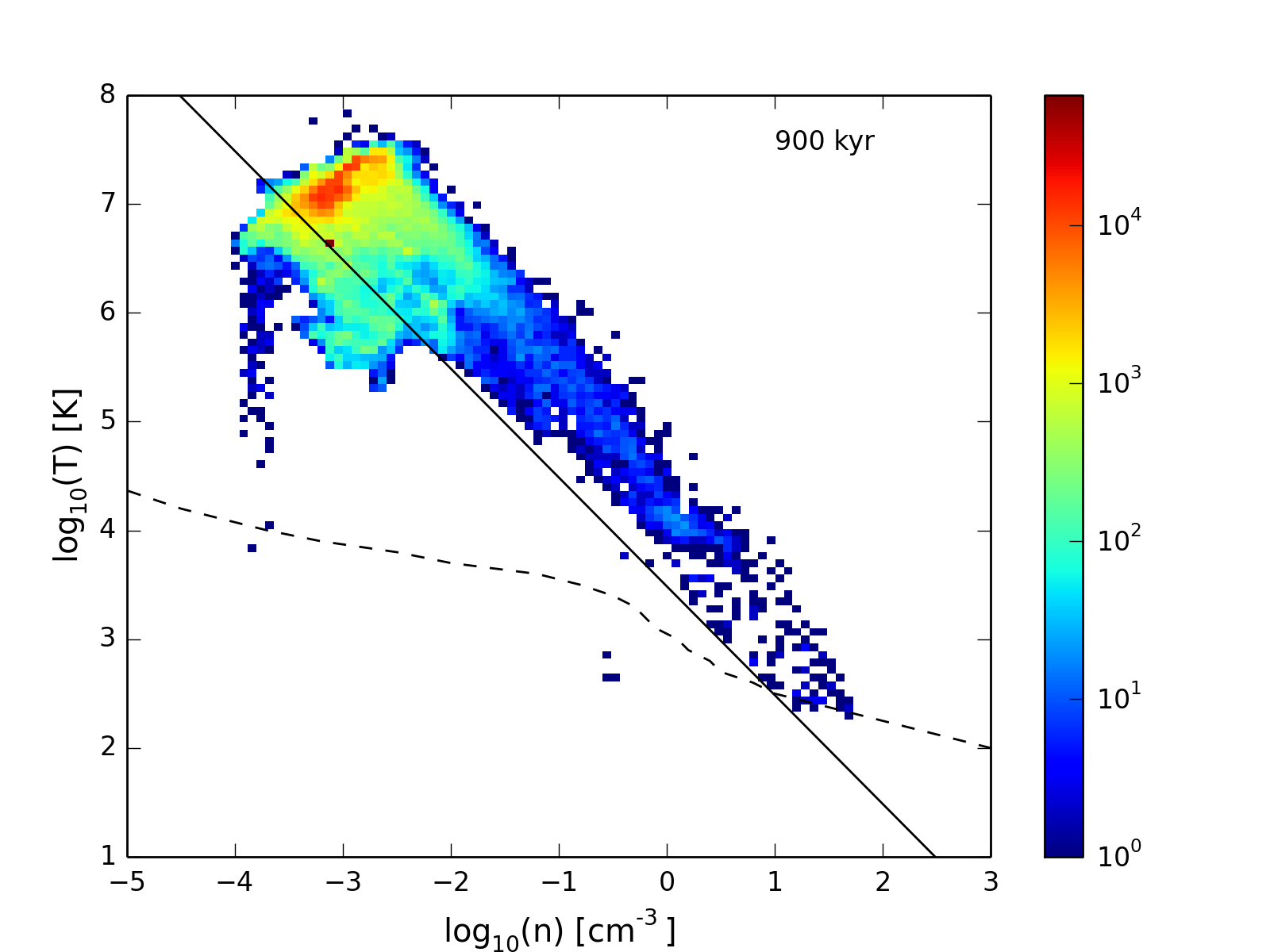Again, we see the offset over-pressurized region. Running in a significantly larger box yields simulations that look okay, but there is still a region of higher pressure.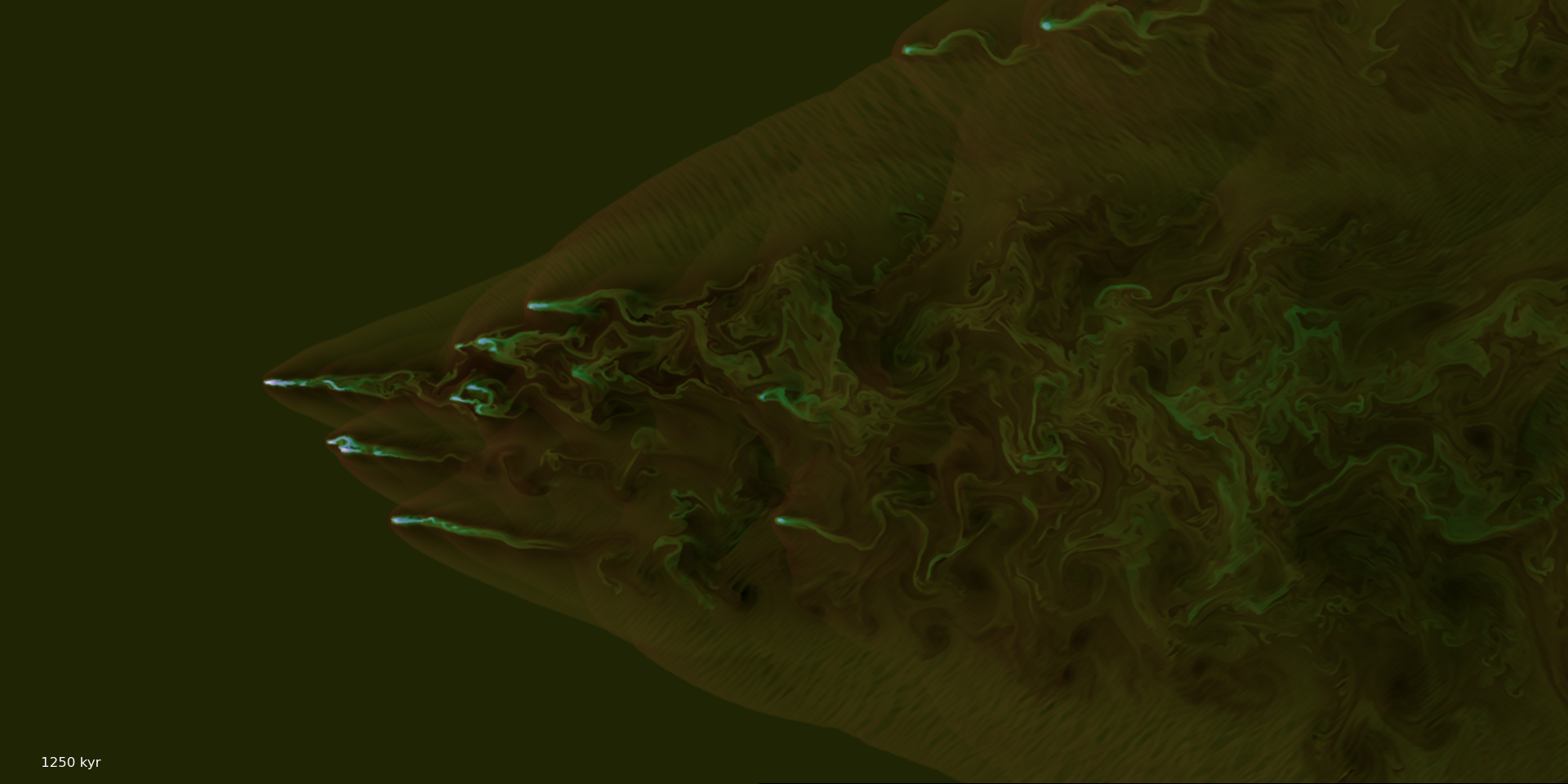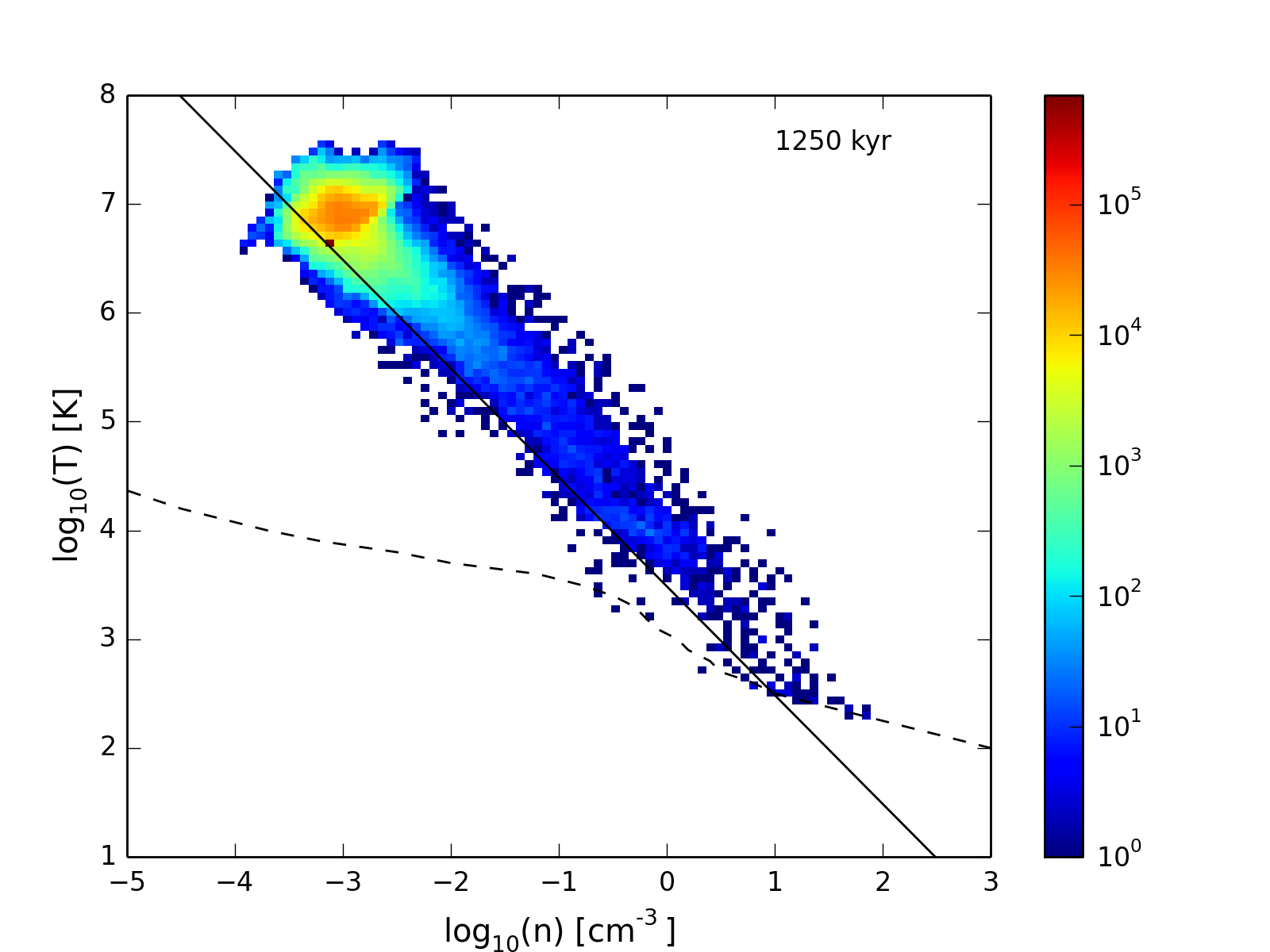This simulation didn’t show any of the odd behavior near the boundary (see this movie). Maybe some of the excess pressure is real, resulting from the interacting bow shocks.

Given these results, how big a box is needed in 3D, for a simulation with $10\times$ higher density? The simulation above has dimensions $300\times120$ pc, but the mach cone has a wider opening angle in 2D. That size box is not big enough for a 2D simulation with $10\times$ higher density: